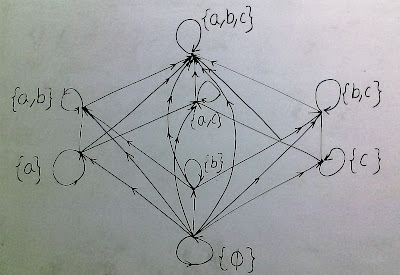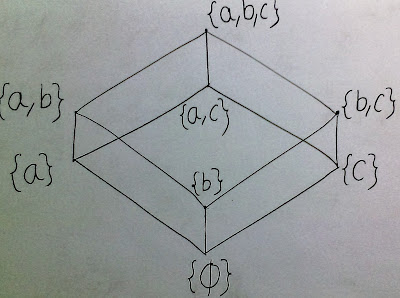Search your topic here

POSET partial order relation

PARTIAL ORDER RELATION

Partial order relation: On a set G, a binary relation R is a partial order relation if R is reflexive, antisymmetric and transitive.

 reflexive xRx antisymmetric xRy implies yRx transitive xRy and yRz implies xRz

POSET

Partial Order Set (Poset):  A set on which partial ordering relation is defined is known as po-set.

HASSE DIAGRAM

Hasse Diagram: Hasse diagram generally used to represent partial order relations in equivalent but in simpler forms by removing non critical parts of the relation.

For example:Below diagram represent a partial order relation.

This can relation can be modify as-
1. Remove all loops.
2. Remove all arrows that can be inferred from transitivity.

Than above diagram represented as-Above diagram is known as Hasse diagram for a partial order relation.

LOWER AND UPPER BOUNDS

Upper Bound:  Let A S, then an element s S is called upper bound of A if and only if-
a s, a A.

Lower Bound: Let A S, then an element s S is called the lower bound of A is and only if-
s a, a A.

Least Upper Bound: If A S and g S, then g is called the least upper bound of A if and only if the following two conditions hold-
a)     g is upper bound of the set A.
b)     g s for every upper bound s of A.
The least upper bound of A is denoted by l.u.b.A or supA.

Greatest Lower Bound: If A S and l S, then l is called the greatest lower bound of A if and only if the following two conditions hold-
a)     l is lower bound of the set A.
b)     s l, for every lower bound s of A.

The greatest upper bound of A is denoted by g.l.b.a. or infA.

LATTICE

Infimum: Infimum is also known as greatest lower bound.

Supremum: Spremum is also known as least upper bound.

LATTICE: A lattice is a po-set (P, ≤) in which every pair of elements has a supremum and an infimum.

Given a, b P, we write these bounds as

a Λ b = inf ({a, b})

a V b = sup({a, b})

Problems based on above study:

Prob. Draw the Hasse Diagram of D60 (divisors of 60).
Solution.

Prob. What is Hasse diagram? Draw the Hasse diagram of the set D30 of positive integral divisor of 30 with relation ‘1’.
Solution.

Prob. Let A = ( a, b, c, d} and P(A) its power set. Draw Hasse diagram of [P(A), ].
Solution.

Prob. Let A = {2, 3, 6, 12, 24, 36} and the relation be such that a ≤ b, if a divides b. Draw the Hasse diagram of (A ≤).
Solution.

Prob. Let L be the set of all factors of 12 and ‘\’ be the divisibility relation on L. Show that (L, ‘\’) is a lattice.
Solution.

Prob. Let L = {1, 2, 3, 4, 6, 8, 9, 12, 18, 24} be ordered by the notation ‘\’ where x/y mean ‘x divides y’. Show that D24 the set of all divisors of the integer 24 of L is a sublattice of the lattice (L, \ ).
Solution.

Prob. If B=Divisors of 24 = (D24) be a lattice then find all the sublattices of D(24). Also draw the Hasse diagram.
Solution.